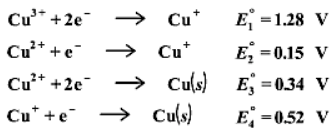Problem: Using the following data, calculate the standard reduction potential for the reaction of Cu(III) to Cu(II).

80% (3 ratings)
Problem Details

Using the following data, calculate the standard reduction potential for the reaction of Cu(III) to Cu(II).